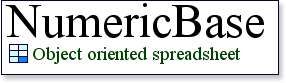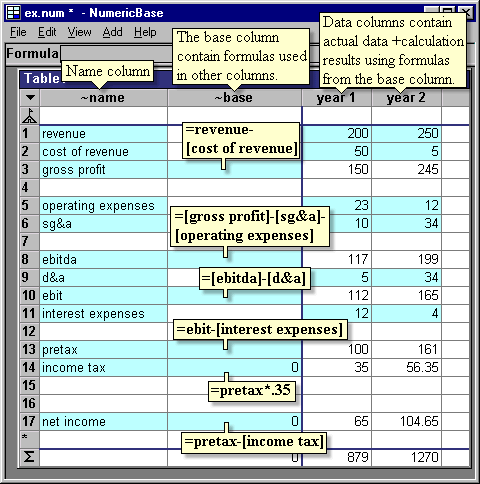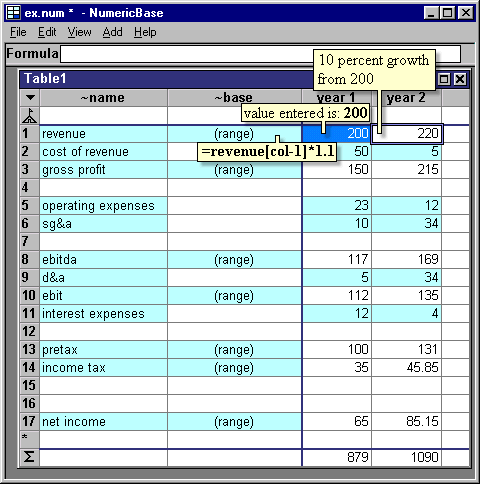# Balance sheet

Balance sheet
The example show a balance sheet for a hypothetical company. It uses the name column to list the different balance items, and the base column to define the calculated items ( gross profit, ebitida, ebit, pretax, income tax, and net income). It is the only column that contain formulas.

The actual data is entered in the data columns( year1, year2), these columns contains only the actual numbers for the specific years, and are using the formulas that we define at the base column to calculate the calculated fields.Screenshot: The balance sheet example

Making predications
The following screenshot is based on the above, with one modification: instead of actual values in the revenue row, we use an assumption that the revenue is growing 10 percent a year, starting with 200. We do this by adding the formula =revenue[col-1]*1.1 at the revenue column and the base column. (The range messages are because the formula is an implicit range)Screenshot: revenue is growing 10 percent a year

The source code
Following is the source code for this example. To use it in your copy of NumericBase, copy and paste it in the View Source window.

table Table1{   ~name:=!   ~base:=!   [year 1]:= !   [year 2]:= !   <1>:={~name:="revenue",~base:=revenue[col-1]*1.1,[year 1]:= 200,}   <2>:={~name:="cost of revenue",[year 1]:= 50,[year 2]:= 5,}   <3>:={~name:="gross profit",~base:=revenue-[cost of revenue],}   <5>:={~name:="operating expenses",[year 1]:= 23,[year 2]:= 12,}   <6>:={~name:="sg&a",[year 1]:= 10,[year 2]:= 34,}   <8>:={~name:="ebitda",~base:=[gross profit]-[sg&a]-[operating expenses],}   <9>:={~name:="d&a",[year 1]:= 5,[year 2]:= 34,}   <10>:={~name:="ebit",~base:=[ebitda]-[d&a],}   <11>:={~name:="interest expenses",[year 1]:= 12,[year 2]:= 4,}   <13>:={~name:="pretax",~base:=ebit-[interest expenses],}   <14>:={~name:="income tax",~base:=pretax*.35,}  <17>:={~name:="net income",~base:=pretax-[income tax],} }
optimized(13,26) result(Table1);
Listing: source code of the balance sheet example